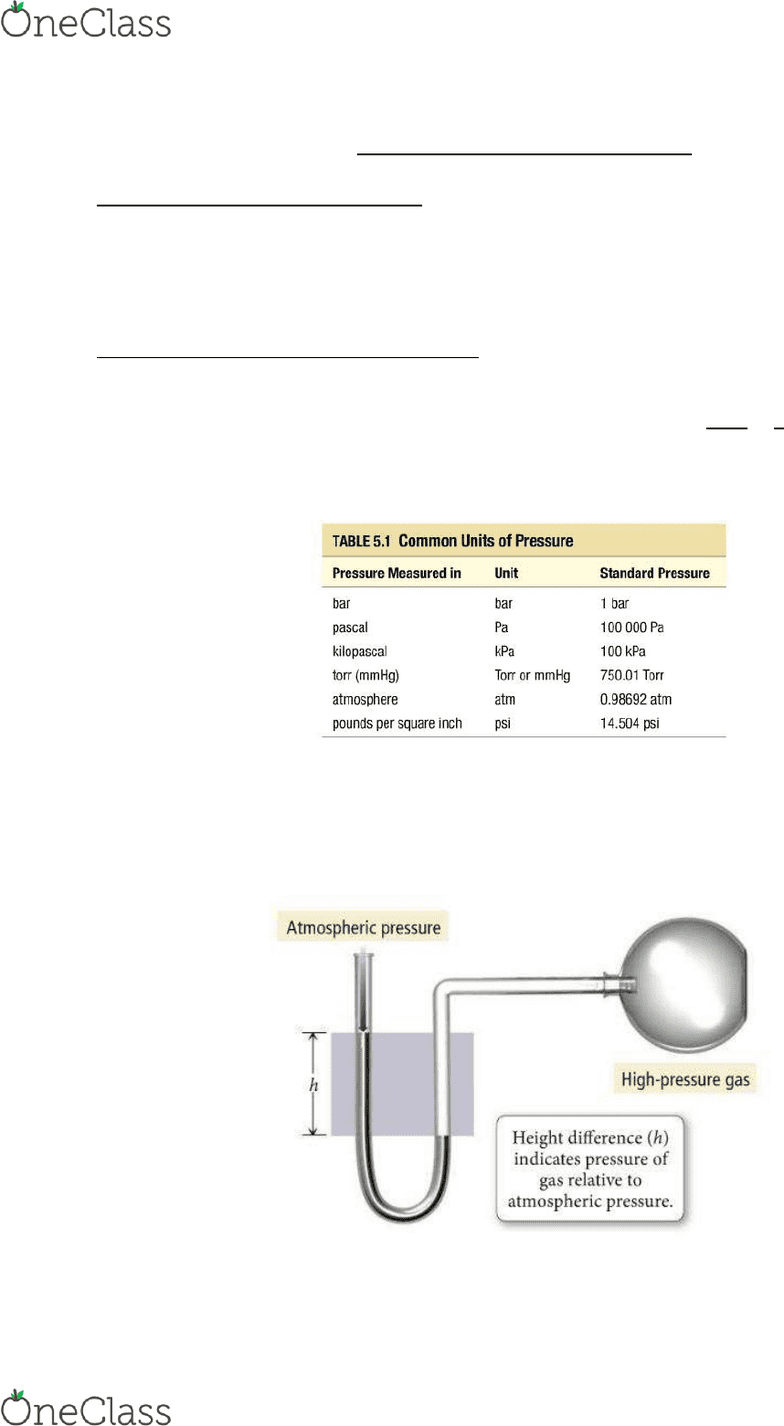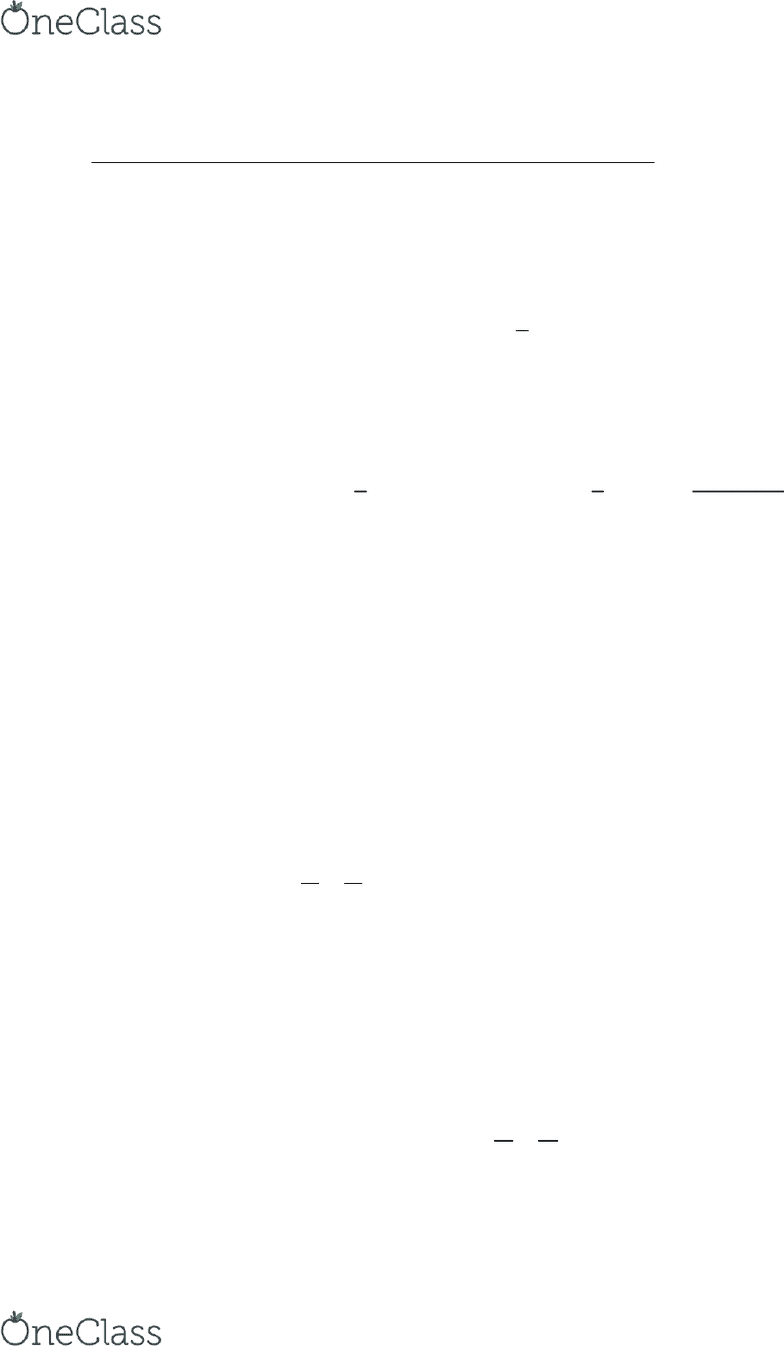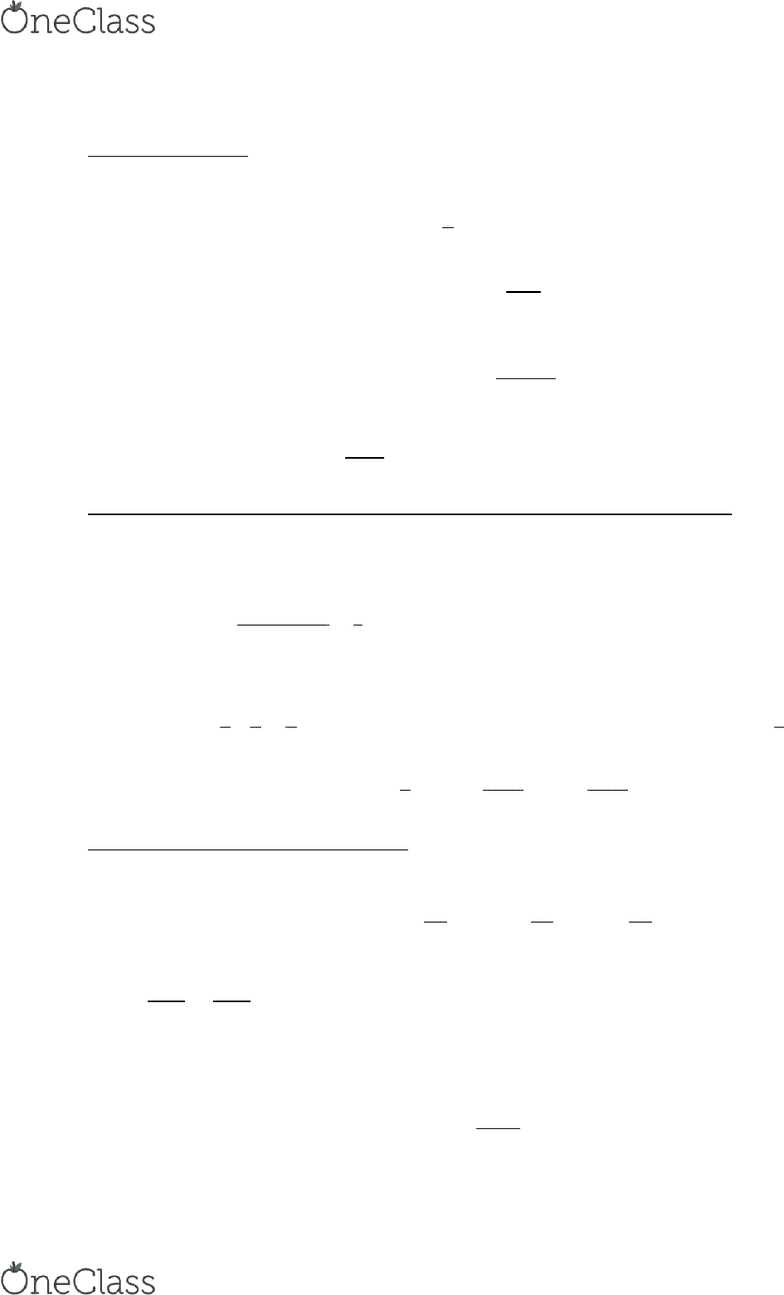Textbook Notes (280,000)
CA (170,000)
Ryerson (10,000)
CHY (90)
CHY 103 (20)
Chapter 5

# CHY 103 Chapter 5: Chemistry Chapter 5 Study Notes

Department
Chemistry
Course Code
CHY 103
Professor
Sharonna Greenberg
Chapter
5

This preview shows pages 1-3. to view the full 9 pages of the document.Mareck Tam CHY 103
Chemistry Chapter 5 Study Notes
5.1 Breathing: Putting Pressure to Work
Gas Pressure: The Force exerted per unit area by gas molecules as they strike the surfaces
around them.
Lower pressure is the result of fewer collisions between molecules and vise versa.
5.2 Pressure: The result of Molecular Collisions
The gas particles collide with each other and with the surfaces around them, and each collision
results in a small force being exerted.
The equation that represents the force of pressure is 
 
A low density of gas results in a low pressure and vise versa for a high density.
The most common pressure unit is millimetre of mercury (mmHg), this originates from how
pressure. A table representing all the units of pressure is presented below:
101 325 Pa = 101.325 kPa = 760 Torr = 1 atm
A way to measure pressure in a laboratory is using a manometer. It is a U shaped tube
containing a dense liquid, usually mercury.
The levels of mercury should be the same on both sides of the tube if the atomospheric pressure
is the same as gas pressure. If atm pressure is higher then the mercury level will be higher on
left side and vise versa if the atm is lower.
find more resources at oneclass.com
find more resources at oneclass.com

Only pages 1-3 are available for preview. Some parts have been intentionally blurred.Mareck Tam CHY 103
5.3 The Siple Gas Las: Boyle’s La, Chales’s La, ad Aagado’s La
The 4 characteristics of gas are: Pressure (P), Temperature (T), Volume (V) and number of moles
(n). When of these values are changed they all are affected.
Boyle’s La: Volue ad Pessue
Volume and Pressure are inversely proportional and when one increases the other decreases.


Follows the idea that pressure results from the collisions of the gas particles with the walls of
their container. When the volume of a gas is decreased, the same number of gas particles is
crowded into a smaller volume. A smaller volume resulting in more collisions with walls and
other particles and therefore the pressure is increased when volume is decreased.
To calculate volume and pressure:



If w wanted to determine the pressure or volume before or after the reaction we can use this
equation:
Chales’s La: Volue ad Tepeatue
The diet popotioality etee Volue ad tepeatue is aed Chales’s La:

When the temperature of a gas sample is increased, the gas particles move faster, collisions
with the walls are more frequent, and the force exerted with each collision is greater. Pressure
can only be constant in a large volume.
To calculate the amount of gas following a temperature change or vise versa, we use this
equation: 
To calculate volumes and temperatures before and after a reaction we can use the following
equation:

Aagado’s La: Volue ad Aout I oles
States that the volume of a gas in directly proportional to the number of moles of gas.

When the amount of gas in a sample increases at a constant temperature and pressure, its
volume increases in direct proportion because the greater number of gas particles fill more
space.
When pressure and temperature are constant we can use the following equation to calculate
the initial and final values of moles and volumes:
find more resources at oneclass.com
find more resources at oneclass.com

Only pages 1-3 are available for preview. Some parts have been intentionally blurred.Mareck Tam CHY 103
5.4 The Ideal Gas Law
The ideal gas law we combine all three laws that we had just learned. Therefore, combining
Aagado’s la, Boyle’s la ad Chales’s la.

When they are combined:
To actually come to an equation instead of the proportionality statement we need to add in a
constant, in this case the ideal gas constant:
Rearranging, we get:
The R constant is 

5.5 Applications of the Ideal Gas Law: Molar Volume, Density, and Molar Mass of a Gas
The volume occupied by one mole of a substance is its molar volume.
The standard temperature is  and pressure is  and is called
STP.


The density can be given by multiplying the molar density my the molar mass.



5.6 Mixtures of Gases and Partial Pressures
The pressure due to any individual component in a gas mixture is the partial pressure (Pn)
Partial Pressures are represented by the following equation:



The sum of all these partial pressures:



The number of moles of a component of a mixture divided by the total number of moles in the
mixture, is called the mole fraction(Xa). Represented the equation by:

find more resources at oneclass.com
find more resources at oneclass.com